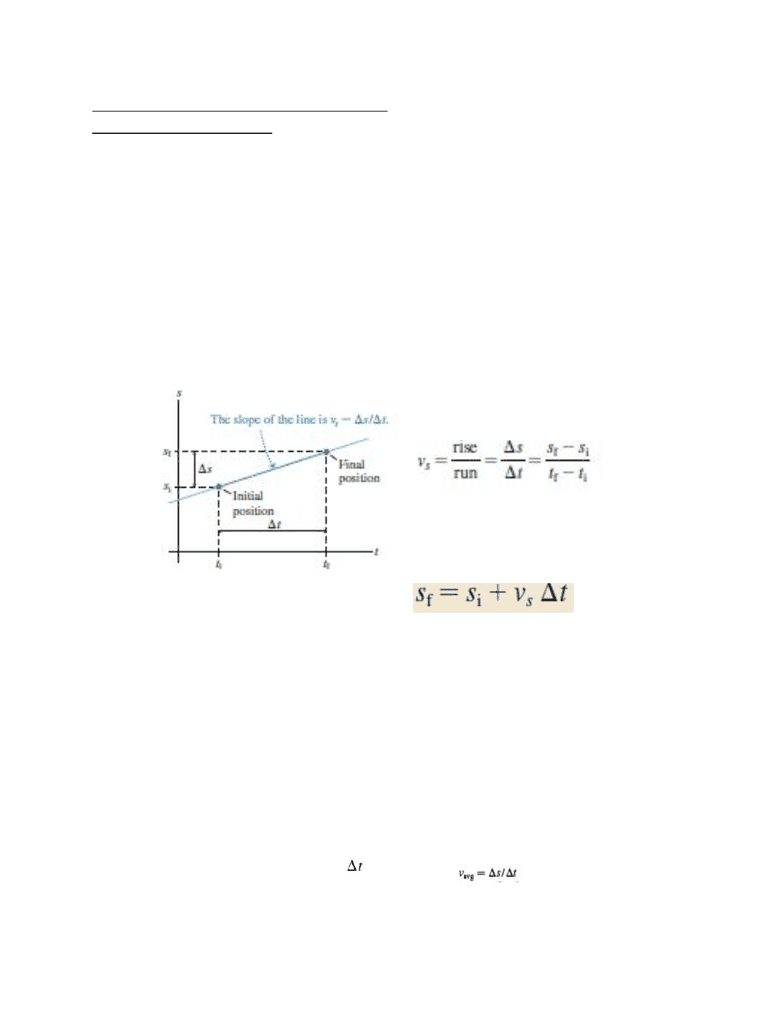Class Notes (1,100,000)
CA (650,000)
UTSC (30,000)
Lecture 4

# PHYA11H3 Lecture Notes - Lecture 4: One Direction, KinematicsPremium

Department
Physics and Astrophysics
Course Code
PHYA11H3
Professor
Gyula Lorincz
Lecture
4

This preview shows half of the first page. to view the full 3 pages of the document.Introduction to Physics: Lecture 4
Physics I for Life Sciences
Chapter 2: Kinematics in One Dimension
Whats is Kinematics?
2.1. Motion in One Dimension
The physics of a object are the same whether it moves along the direction
of the x-axis or the y-axis.
To model this, take a position graph as shown below. The initial position
of the object at time ti is si and its final position  is sf at time tf.
To find the velocity of the
object, you must find the rise/run of the
line. Mathematically shown as :
By rearranging the above
equation, we arrive at one of the
fundamental equations of kinematics for
motion in one dimension:
2.2. Instantaneous Velocity
Uniform motion is a very simplified version of the motion that happens in
the real world. Rarely will you find an object moving at a constant velocity
for long periods of time.
As seen from previous position graphs, when velocity increases the slope
of the line also increases, causing an upwards curving graph.
Instantaneous velocity: defined as the velocity (speed and direction) of
an object at a instant of time.
Instantaneous velocity is derived using concepts from calculus. The
equation for average velocity is used to approximate velocity at an exact
moment of time as the in the equation shown